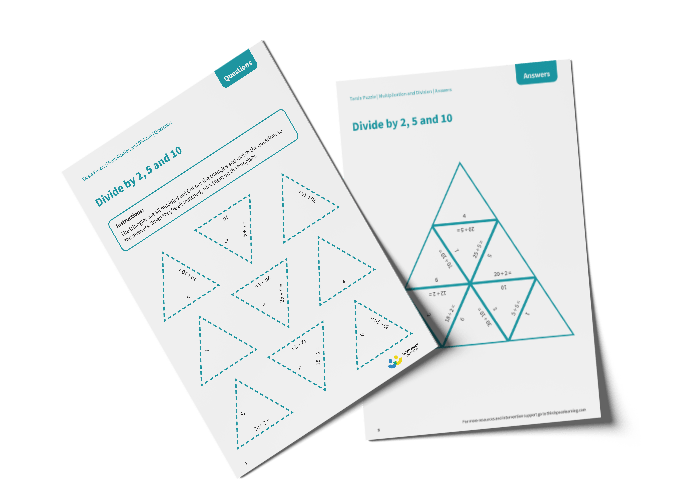# Tarsia Puzzle Divide by 2, 5 and 10 (Year 2)

The triangles in the tarsia puzzle have all been mixed up. In this dividing by 2, 5 and 10 tarsia puzzle, pupils will need to match the division question to the correct answer to create one large triangle.

This Year 2 divide by 2, 5 and 10 tarsia puzzle worksheet contains:

• One question sheet
• One template sheet

Year 2

Division